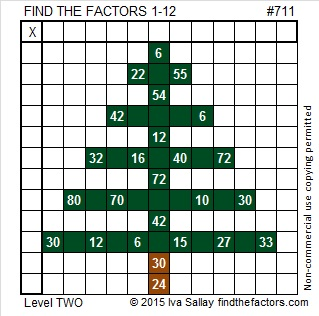# Could 711 be Eleven 7 in base 64?

• 711 is a composite number.
• Prime factorization: 711 = 3 x 3 x 79, which can be written 711 = (3^2) x 79
• The exponents in the prime factorization are 2 and 1. Adding one to each and multiplying we get (2 + 1)(1 + 1) = 3 x 2  = 6. Therefore 711 has exactly 6 factors.
• Factors of 711: 1, 3, 9, 79, 237, 711
• Factor pairs: 711 = 1 x 711, 3 x 237, or 9 x 79
• Taking the factor pair with the largest square number factor, we get √711 = (√9)(√79) = 3√79 ≈ 26.66458.Here is today’s puzzle:Print the puzzles or type the solution on this excel file: 12 Factors 2015-12-14

———————————————————————————

Even though in English 711 is a rather fun rhyming number, it has been difficult for me to find interesting facts about it:

7 + 1 + 1 = 9 so 711 is divisible by both 3 and 9.

It can be written as the sum of consecutive numbers three ways:

• 355 + 356 = 711; that’s two consecutive numbers.
• 236 + 237 + 238 = 711; that’s three consecutive numbers.
• 75 + 76 + 77 + 78 + 79 + 80 + 81 + 82 + 83 = 711; that’s nine consecutive numbers.

Is 711 a palindrome in any other base? Not in any base less than 78, but in BASE 78 it is palindrome 99 because 9(78) + 9(1) = 711.

We could ALMOST write that 711 is 11 7 in BASE 64 because 11(64) + 7(1) = 711. However, we can’t REALLY write that because base 10’s eleven would most likely be represented by the letter B in base 64. Thus we would likely write 711 as B7 in Base 64.

Since the words “eleven” and “twelve” do not contain any reference to the word “ten”, I suppose in English we could say that 711 is eleven 7 in base 64. We likely wouldn’t be able to make that same claim in other languages, however.

————————————————————————————This site uses Akismet to reduce spam. Learn how your comment data is processed.